• 按条件取数VBA

2018-04-21 17:09:36
EXCEL用的vba程序可以用来进行条件统计取数计算等等。
• 我们利用数据库，主要的目的是存储数据，在需要的时候可以提取出来，提取的同时可以对数据进行必要的处理，本讲就是讲解从数据库中多条件提取数据的方法。实例：我们有如下的数据库数据资料，要在其中提取出我们的...

大家好，今日我们讲VBA解数据库解决方案的第49讲内容：从数据库中，多条件提取出数据的方法。我们利用数据库，主要的目的是存储数据，在需要的时候可以提取出来，提取的同时可以对数据进行必要的处理，本讲就是讲解从数据库中多条件提取数据的方法。

实例：我们有如下的数据库数据资料，要在其中提取出我们的数据：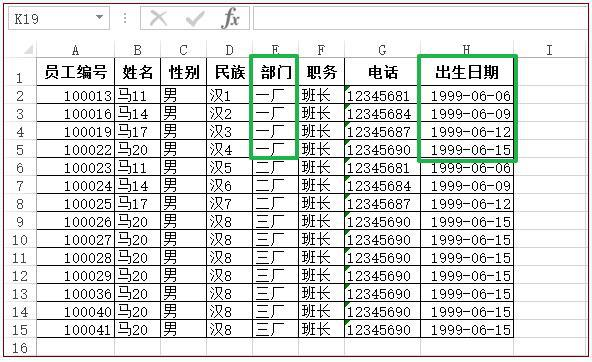我们的目的是在其中提取出“部门”为“一厂” 并且“职务”为“班长”，同时“出生日期”在1999/6/9或之后的员工记录，该怎么解决呢？

我们分析一下：上述的要求是三个查询条件，我们可以整合在SQL语句中实现。

下面看我给出的代码：

Sub mynzRecords_49() '第49讲 从数据库中，多条件提取数据的方法

Dim strPath, strSQL, strTable As String

strPath = ThisWorkbook.Path & "\mydata2.accdb"

strTable = "员工信息"

'多条件提取数据的SQL语句结构

strSQL = "select * from " & strTable _

& " where 部门='一厂' and 职务='班长' and 出生日期>=#1999/6/9#" & " ORDER BY 员工编号 DESC"

Worksheets("49").Select

Cells.ClearContents

For i = 0 To rsADO.Fields.Count - 1

ActiveSheet.Cells(1, i + 1) = rsADO.Fields(i).Name

Next i

For i = 1 To rsADO.RecordCount

For j = 0 To rsADO.Fields.Count - 1

ActiveSheet.Cells(i + 1, j + 1) = rsADO.Fields(j)

Next j

Next i

.Font.Bold = True

.HorizontalAlignment = xlCenter

End With

Columns.AutoFit

End Sub

代码截图：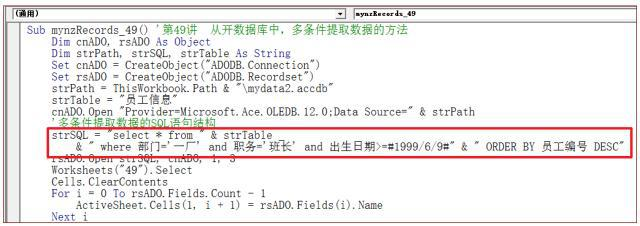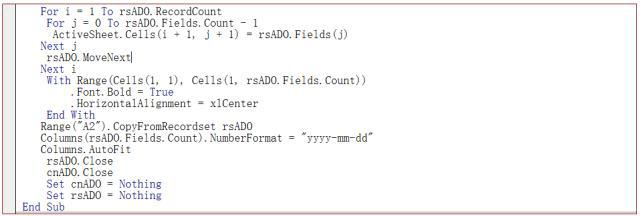代码解析：

1 '多条件提取数据的SQL语句结构

strSQL = "select * from " & strTable _

& " where 部门='一厂' and 职务='班长' and 出生日期>=#1999/6/9#" & " ORDER BY 员工编号 DESC"

在上面的代码中我们实现了提取出“部门”为“一厂” 并且“职务”为“班长”，同时“出生日期”在1999/6/9或之后的员工记录，其中

① "select * from " & strTable 是取数据库的所有字段

② " where 部门='一厂' 是部门的条件

③ and 职务='班长' 是条件职务的要求

④ and 出生日期>=#1999/6/9#" 是对出生日期的要求

⑤ ORDER BY 员工编号 DESC" 是对员工编号 的降序排列

注意点：中间的and 是连接各个条件的逻辑语言，## 是日期符号的表述

仍是借助于工作表条件格式的设定完成对出生日期的显示

下面看代码的运行结果：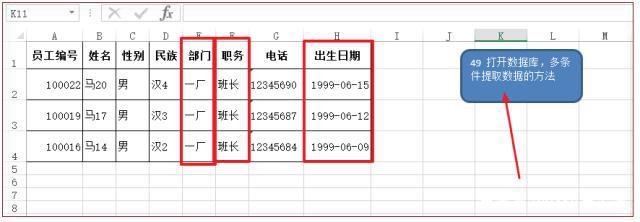今日内容回向：

1 如何实现多条件数据的提取？

2 数据的日期格式是如何表现的？

展开全文• 在已经输入的数据中，找到并复制想要的数据，然后粘贴到指定的地方，是再自然不过的操作了。或者从工作的一个单元格区域复制到同一工作中另外的单元格区域，或者从工作的一个单元格区域复制到另一工作中的...

学习Excel技术，关注微信公众号：

excelperfect

在Excel工作表中，复制粘贴是最常用的操作之一。在已经输入的数据中，找到并复制想要的数据，然后粘贴到指定的地方，是再自然不过的操作了。或者从工作表的一个单元格区域复制到同一工作表中另外的单元格区域，或者从工作表的一个单元格区域复制到另一工作表中的单元格区域，甚至从工作表的一个单元格区域复制到不同工作簿中的工作表单元格区域。那么，如何使用VBA代码来实现复制粘贴操作呢？本文将介绍常用的一些代码。

直接赋值

如下图1所示，使用代码：

Range("D1:E2").Value= Range("A1:B2").Value

将单元格区域A1:B2中的值直接复制到单元格D1:E2中。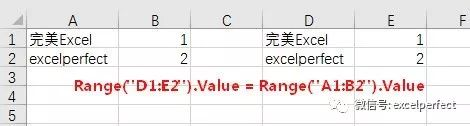图1

使用Copy方法

也可以使用Copy方法，将单元格区域A1:B2中的值复制到以单元格D1开头的单元格区域中：

Range("A1:B2").CopyRange("D1")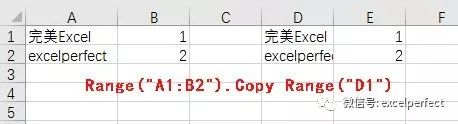图2

使用数组

如下图3所示，将工作表Sheet4的列A中内容为“完美Excel”的行复制到工作表Sheet5中。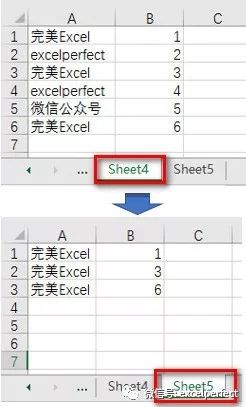图3

可以使用下面的代码：

Sub CopyDataByArray()

Dim arr As Variant

Dim i As Long

Dim j As Long

Dim row As Long

row = 1

arr =Sheet4.Range("A1").CurrentRegion.Value

For i = LBound(arr) To UBound(arr)

If arr(i, 1) = "完美Excel" Then

For j = LBound(arr, 2) ToUBound(arr, 2)

Sheet5.Cells(row, j).Value =arr(i, j)

Next j

row = row + 1

End If

Next i

End Sub

代码中，将工作表Sheet4中的数据存储到数组中。然后，判断数组中第1维的值是否为“完美Excel”并复制到工作表Sheet5中。注意，数组变量必须声明为Variant型。

使用For循环

使用For循环，也可以实现上图3的结果。代码如下：

Sub CopyDataByFor()

Dim rng As Range

Dim i As Long

Dim j As Long

Dim row As Long

Set rng = Sheet4.Range("A1").CurrentRegion

row = 1

For i = 1 To rng.Rows.Count

If rng(i, 1).Value = "完美Excel" Then

For j = 1 To rng.Columns.Count

Sheet5.Cells(row, j).Value =rng(i, j).Value

Next j

row = row + 1

End If

Next i

End Sub

使用自动筛选

使用自动筛选，不必使用很多次循环，也能实现上图3所示的结果。代码如下：

Sub CopyDataByAutoFilter()

Dim rng As Range

Set rng = Sheet4.Range("A1").CurrentRegion

'删除已存在的筛选

rng.AutoFilter

'应用自动筛选

rng.AutoFilter Field:=1, Criteria1:="完美Excel"

'复制数据

Sheet4.Range("A1").CurrentRegion.SpecialCells(xlCellTypeVisible).Copy

Sheet5.Range("A1").PasteSpecialxlPasteValues

'删除筛选

rng.AutoFilter

End Sub

使用高级筛选

高级筛选能够直接将满足条件的数据复制到指定的位置，但需要先指定条件。如下图4所示，工作表Sheet10中的单元格区域A1:B7为数据区域，单元格区域D1:D2为筛选条件，需要筛选出名称为“完美Excel”的数据至工作表Sheet11中。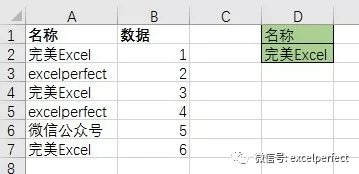图4

代码如下：

Dim wksData As Worksheet

Dim wksFilter As Worksheet

Dim rngData As Range

Dim rngCriteria As Range

Set wksData =ThisWorkbook.Worksheets("Sheet10")

Set wksFilter =ThisWorkbook.Worksheets("Sheet11")

'清空要放置复制数据的工作表

wksFilter.Cells.Clear

'删除已存在的筛选

If wksData.FilterMode = True Then

wksData.ShowAllData

End If

'获取数据区域

Set rngData =wksData.Range("A1").CurrentRegion

'条件区域

Set rngCriteria =wksData.Range("D1:D2")

'筛选并获取满足条件的数据

CriteriaRange:=rngCriteria, _

CopyToRange:=wksFilter.Range("A1")

End Sub

运行代码后的结果如下图5所示。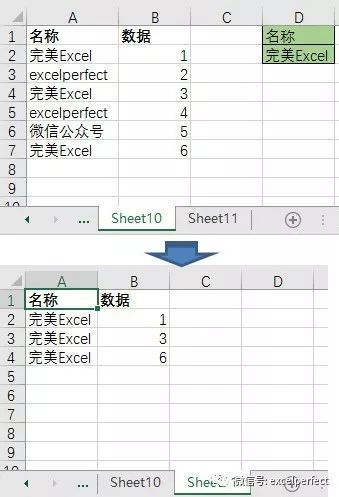图5

高级筛选还可以处理多个条件，对于同一行中的条件关系为“AND”，对于不同行中的条件关系为“OR”。

提示

1. 在使用VBA代码进行复制操作时，我们不需要先选择想要复制的数据，也不需要选择或激活数据所在的工作表。

2. 在不同的工作表之间复制，或者在不同的工作簿之间复制时，在前面加上相应的工作表或工作簿名称。

3. 在复制前关闭Excel的某些功能，可以加速复制操作。一般，在复制代码前，使用下面的代码关闭相关的功能：

Application.Calculation =xlCalculationManual

Application.DisplayStatusBar =False

Application.EnableEvents =False

Application.ScreenUpdating =False

在复制代码完成后，再恢复相关的功能：

Application.Calculation =xlCalculationAutomatic

Application.DisplayStatusBar =True

Application.EnableEvents = True

Application.ScreenUpdating =True

相关文章链接：

Excel VBA解读(49)：复制或剪切单元格——Copy方法与Cut方法

Excel VBA解读(52)：自动筛选方法——AutoFilter方法展开全文• VBA-自动筛选符合条件的数据

千次阅读 2020-03-09 20:20:11
2.我们可以看到符合条件---即入住日期刚好满7天的数据信息被筛选出来 3.代码如下 Option Explicit Dim w0 As Workbook Dim book0 As Worksheet Dim book1 As Worksheet Dim r0 As Range Dim r1 As Range Sub 自动...

1.效果图如下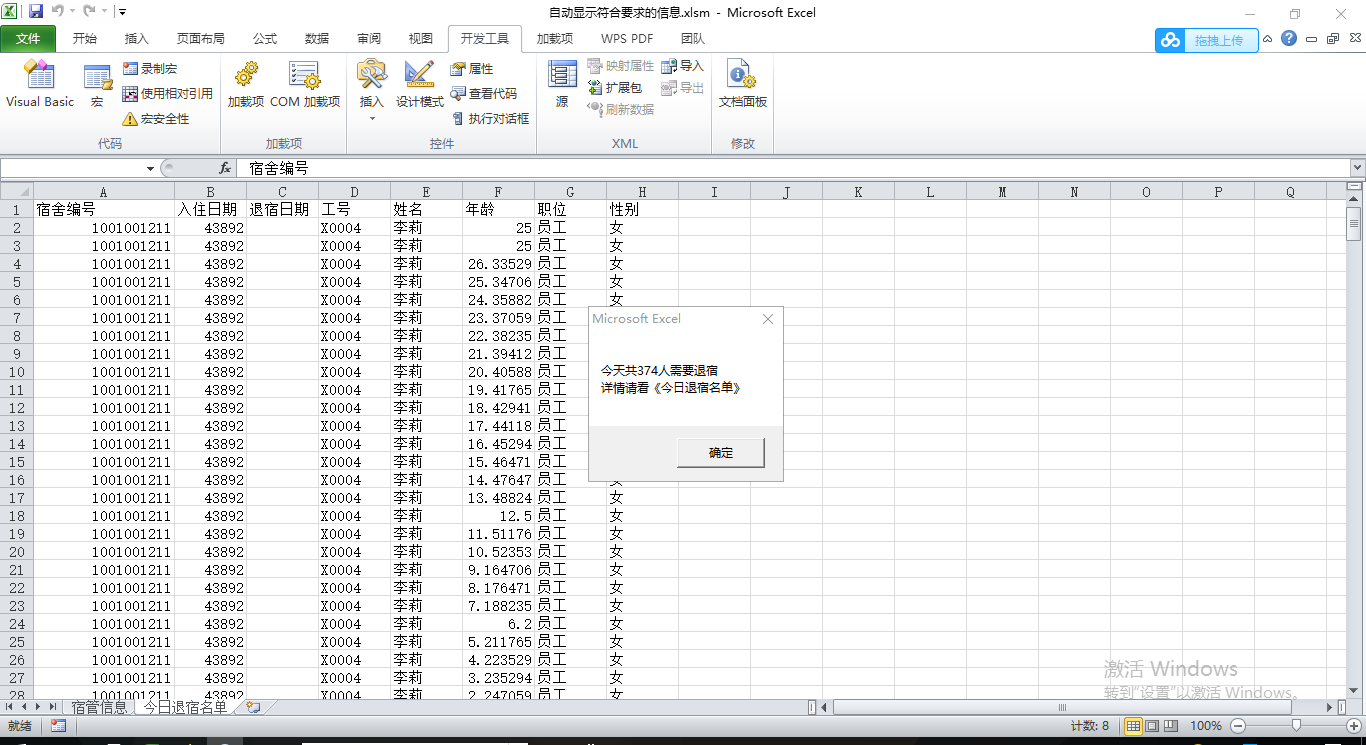2.我们可以看到符合条件---即入住日期刚好满7天的数据信息被筛选出来

3.代码如下

Option Explicit
Dim w0 As Workbook
Dim book0 As Worksheet
Dim book1 As Worksheet
Dim r0 As Range
Dim r1 As Range
Sub 自动筛选符合条件的信息()
Set w0 = ActiveWorkbook
Set book0 = w0.Worksheets("宿管信息")
Set book1 = w0.Worksheets("今日退宿名单")
Set r0 = book0.UsedRange
Set r1 = book1.UsedRange
Dim i As Long
Dim j As Integer
Dim indlenth As Integer
Dim aim()
Dim count2 As Long
Dim k As Long
book1.Cells.Clear
'将原始数据写入数组
Dim ori()
Dim needleave As Range
ori = r0
indlenth = 1
Do While indlenth <= UBound(ori, 2) - 1
If ori(1, indlenth) = "入住日期" Then Exit Do
indlenth = indlenth + 1
Loop
'将表头复制
r0.Resize(1, r0.Columns.count).Copy
book1.Select
Cells(1, 1).Select
ActiveSheet.Paste
'判断是否属于需要退宿人员（满足条件）
k = 1
'运用公式将满足条件的数据条数计算出来
count2 = Application.WorksheetFunction.CountIf(r0.Resize(r0.Rows.count, 1).Offset(0, indlenth - 1), Date - 7)
ReDim aim(1 To count2, 1 To UBound(ori, 2))
For i = 2 To UBound(ori, 1)
Set needleave = r0.Resize(1, r0.Columns.count)
'将满足条件的信息明细赋值给目标数组
If Date - CDate(ori(i, indlenth)) = 7 Then
For j = 1 To UBound(ori, 2)
aim(k, j) = ori(i, j)
Next
k = k + 1
End If
Next
Dim finally As Range
Set finally = r1.Resize(count2, UBound(ori, 2)).Offset(1, 0)
finally = aim
If count2 > 0 Then
MsgBox ("今天共" & count2 & "人需要退宿" & Chr(10) & "详情请看《今日退宿名单》")

Else
MsgBox ("今天无人退宿！")
End If
End Sub

展开全文• VBA提取指定区间的数据

千次阅读 2020-04-12 19:14:53
Sub OptionalFeatureLicense() Dim Fs As Object, Ft As Object, S As String Set Fs = CreateObject("Scripting.FileSystemObject") Set d = CreateObject("scripting.dictionary") filePath = Th...

Dim Fs As Object, Ft As Object, S As String
Set Fs = CreateObject("Scripting.FileSystemObject")
Set d = CreateObject("scripting.dictionary")
filePath = ThisWorkbook.Path & "\data\"
fileName = Dir(filePath & "*.log", vbNormal)
' n = 1
X = 0
Do While fileName <> ""
Set Fs = CreateObject("Scripting.FileSystemObject")
Set Ft = Fs.opentextfile(filePath & fileName)
Do
If InStr(1, texTline, "MO ") > 0 And InStr(1, texTline, "OptionalFeatureLicense=") > 0 Then

X = X + 1
' n = n + 1
STRN = Split(Application.Trim(texTline), " ")
Count = UBound(STRN)
'Worksheets("SHEET1").Cells(n, 1) = fileName
'Worksheets("SHEET1").Cells(n, X + 1) = STRN(1)

Do

If InStr(1, texTline, "==") > 0 Then
Else:

STRN = Split(Application.Trim(texTline), " ")
Count = UBound(STRN)
If Count >= 1 Then
For J = 1 To Count
S = S & " " & STRN(J)
Next
'X = X + 1
'Worksheets("SHEET1").Cells(n, X + 1) = S
S = ""
End If
End If

Loop Until InStr(1, texTline, "==") > 0

End If
If d.Count > 0 Then
'For K = 0 To d.Count - 1
'Worksheets("SHEET1").(X+1,K) = Application.Transpose(d.keys)
' L1 = d.KEYS
'L2 = d.ITEMS
'Worksheets("sheet1").Cells(X, 1 + K) = L1(K)
'Worksheets("sheet1").Cells(X, 1 + K) = L2(K)
'Next
For K = 1 To Application.CountA(Worksheets("featureState").Rows(1))
L1 = d.KEYS
L2 = d.ITEMS
Worksheets("OptionalFeatureLicense").Cells(X + 1, K) = d(o)
Next
End If
d.RemoveAll

'X = 0
'Loop Until InStr(1, texTline, ">>> Total") > 0
Loop Until Ft.atendofstream 'Ft.atendofline 'Ft.AtEndOfLine
Set Fs = Nothing
Set Ft = Nothing
Close #1
fileName = Dir
Loop
MsgBox ("已完筛选、合并操作!")
End Sub

展开全文• Excel VBA 根据Sheet2中的表格数据处理Sheet1中的数据，包括：1，对sheet1和sheet2指定数据的循环比较；2，符合条件数据复制至sheet1中，并且用表格颜色进行标记Excel VBA
• 在一个工作簿中,想对某一列做筛选,并且拆分到后面的工作里,通用代码如下: Sub chaifenshuju() Dim sht As Worksheet Dim k, i, j As Integer Dim irow As Integer '这个说的是一共多少 Dim l As Integer Dim sht...
• k + 1) = arr2(i) Next Sheets("数据提取").Select Application.ScreenUpdating = True End Sub Sub cls() Application.DisplayAlerts = False Range("a1:j10000").Clear For i = Sheets.Count To 1 Step -1 If ...
• 俺也不是VBA学员，只得从网上临时学习VBA知识，以解其燃眉之急！现将心得体会与大家分享，虽然不是啥好方法，但是足以应付她的问题了，凡事只可问心无愧，本文绝无炫耀之意；希望对大家有所帮助！注：图片中显示的...
• 如何使用VBA比较两张的编号与数量，并建立一张对照，分别列出两张的不同：编号同时存在但数量不同；编号在其中一张中存在，而另一张中不存在（标注哪张表不存在）? 示例数据表 1 编号 名称 ...
• 2 跨取其他工作内容的问题和解决 2.1 总结下问题 引用其他工作，路径问题 操作前需要先打开的问题 打开其他工作，在前台，和后台，避免被打断的问题   2.2 问题1:如何取其他工作簿的名字 ...
• Sub 提取部分CSV数据() Dim FileName As String Dim txtLine As String FileName = ThisWorkbook.Path & "\EUtranCellRelation.csv" Open FileName For Input As #1 Open ThisWorkbook.Path & "\output.CSV...
• 莫以小善而不为，莫以恶小而为之。心善则美，心纯则真。怀善心，做善事，一生无愧。怀平常心，做平常事，日夜安宁。把浮躁的心静下来，去感悟真实的时间，静下心，常存感恩...逆境时学习，积累的就是福报，学习...
• Sub d1() Dim arr, arr1(1 To 1000, 4) arr = Range("a1:d10") Dim x, k For x = 1 To UBound(arr) If arr(x, 1) = "B" Then k = k + 1 arr1(k, 1) = arr(x, 1) arr1(k, 2) = arr(x, 2)win10 vba excel 数据
• 本工作使用VBA实现了如下功能： 1、实时统计重复项 2、重复项数据自动求和 附上代码 Sub lqxs() Dim Cnn As Object, SQL$, shnm$, arr, i&, j& Set Cnn = CreateObject("Adodb.Connection") shnm...excel vba 统计模型 数据分析
• 我想通过从另一个Excel工作“效率”中提取数据，在一个Excel工作“Ship”上构建一个 . “效率”上的数据“发货”，“离开”，“导入”和“导出”进行分类 . 每个类别(装运，休假，进口，出口)都有几个...java表格选择的行
• 数据匹配之VBA

千次阅读 2020-06-16 18:56:33
1. 什么是VBA？ Visual Basic for Applications（简称VBA）是新一代标准宏语言，是基于Visual Basic for Windows 发展而来的。它与传统的宏语言不同，传统的宏语言不具有高级语言的特征，没有面向对象的程序设计概念...excel vba
• 程序功能： 判断excel文件中某个工作簿中第一列的最后一行数据的行数（假设第一数据，以后每行数据为连续的，无空值。）
• 如上图，想在数据透视中只显示红色区域的内容，手动勾选就比较繁琐。 实现思路： 先复制红色的内容。 鼠标停留在数据透视【型号】列的任意数据上（通过该单元格可以获取数据透视和字段） 由于数据透视...
• 前面两篇博客，我们介绍了VBA和使用VBA获取当前工作和另一个工作簿的工作中的数据。这篇我们来说说如何使用VBA模糊查找当前工作中有用的数据。我们有时会遇到这样的情况。我们手头有很老的excel文件，...实例 vba excel
• 笔者最近在做一个数据库项目，其中需要从EXCEL中提取关键字段。提取内容如下图所示，需要将图中加粗部分单独提出后进行去噪处理。如果通过word处理，文字量小的时候尚可实现，但几十万字的处理量很容易就造成假死。...
• 多条件查询并返回数据，是在数据查询时经常会使用的功能，利用字典与数组结合，能够高效地实现此功能。数据源图如下。 现需要根据款号，编号两个条件，查询工艺、数量、报价信息，示例代码如下。 Function ...
• 本人想从个EXCLE数据表中查询数据结果，想用EXCLE公式或者VBA实现，但是技术入门才，请教各位技术人才，帮写下 1.数据表EXCLE个（这里做了3个，实际可能成百上千），名称规范统一命名的（和汇总表数据里名称列...windows
• 实例需求：待处理字符串如下，需要提取其中包含“电子”的部分，即：电子计算机、微型电子管、新创电子 -电脑-电脑硬盘-电子计算机-晶体管-微型电子管-新创电子- 示例代码如下。 Sub Demo1() Dim strWord As ...正则表达式 正则 提取数据 关键字 正则匹配
• 每天一篇Excel技术图文微信公众号：Excel星球NO.670-指定分隔符分段取数据作者：看见星光微博：EXCELers / 知识星球：ExcelHI，大家好，我是星光。打个响指，出一道练习题。如下图所示，C列为数据源，有些数据以"/...R count函数
• 要求：若A列满足值为c，则将b列对应的内容背景色调为红色，并提取出来： 代码如下： Sub naqu()Dim i As IntegerFor i = 2 To Range("a65536").End(xlUp).Row If Cells(i, 1) = "c" Then n = n + 1 Cells(i,...
• 4) = rs("cardtype") sht.Cells(i, 5) = rs("sktime") sht.Cells(i, 6) = rs("gatime") rs.MoveNext '把指针移向下一条记录 i = i + 1 Loop rs.Close cn.Close End Sub 上面这两排是从数据库中取出来的数据，左边...
• 怎么用Java读取两个Excel里的数据并进行可以用GCExcel这个组件，GCExcel涵盖了几乎所有的Excel基本操作，并且可以无损导入/导出 Excel ，包括数据透视、图表、注释、条件格式、数据验证、公式、形状、图片、迷你图...
• 在Excel表格中说起数据信息类别拆分，很朋友都会想到利用数据透视显示报表筛选页的功能或者使用VBA代码。这篇文章为朋友们分享一种使用函数实现数据拆分的方法。一.实例要求：把下图所示的名称为“信息”的......